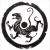## 利用 Java 实现组合式解析器

DSL 设计基础

• 原子：语言中最简单、最基本的实体；
• 组合手段：把原子组合起来构成更复杂实体的方法；
• 抽象手段：命名复杂实体的方法，命名后的复杂实体可以和原子一样通过组合手段组合成为更复杂的实体。

 `interface Parser { public Result parse(String target); } `

 `class Result { private String recognized; private String remaining; private boolean succeeded; private Result(String recognized, String remaining, boolean succeeded) { this.recognized = recognized; this.remaining = remaining; this.succeeded = succeeded; } public boolean is_succeeded() { return succeeded; } public String get_recognized() { return recognized; } public String get_remaining() { return remaining; } public static Result succeed(String recognized, String remaining) { return new Result(recognized, remaining, true); } public static Result fail() { return new Result("", "", false); } } `

 `class Zero implements Parser { public Result parse(String target) { return Result.succeed("", target); } }`

Zero 解析器一定会解析成功，不做任何语法单位识别并直接返回目标字符串。下面我们来定义另外一个很简单的解析器 Item，只要目标字符串不为空，Item 就会把目标字符串的第一个字符作为其识别结果，并返回成功，如果目标字符串为空，就返回失败，Item 的定义如下：

 `class Item implements Parser { public Result parse(String target) { if(target.length() > 0) { return Result.succeed(target.substring(0,1), target.substring(1)); } return Result.fail(); } } `

Zero 和 Item 是我们解析器 DSL 中仅有的两个原子，在下一小节中，我们来定义解析器的组合方法。

 `interface Predicate { public boolean satisfy(String value); } class SAT implements Parser { private Predicate pre; private Parser parser; public SAT(Predicate predicate, Parser parser) { this.pre = predicate; this.parser = parser; } public Result parse(String target) { Result r = parser.parse(target); if(r.is_succeeded() && pre.satisfy(r.get_recognized())) { return r; } return Result.fail(); } } `

 `class IsDigit implements Predicate { public boolean satisfy(String value) { char c = value.charAt(0); return c>='0' && c<='9'; } }`

 `Parser digit = new SAT(new IsDigit(), new Item()); `

 `class OR implements Parser { private Parser p1; private Parser p2; public OR(Parser p1, Parser p2) { this.p1 = p1; this.p2 = p2; } public Result parse(String target) { Result r = p1.parse(target); return r.is_succeeded() ? r : p2.parse(target); } }`

 `class IsAlpha implements Predicate { public boolean satisfy(String value) { char c = value.charAt(0); return (c>='a' && c<='z') || (c>='A' && c<='Z'); } } `

 `Parser alpha = new SAT(new IsAlpha(), new Item()); `

digit_or_alpha 解析器定义：

 `Parser digit_or_alpha = new OR(digit, alpha); `

 `public static Result concat(Result r1, Result r2) { return new Result( r1.get_recognized().concat(r2.get_recognized()), r2.get_remaining(), true); } `

 `class SEQ implements Parser { private Parser p1; private Parser p2; public SEQ(Parser p1, Parser p2) { this.p1 = p1; this.p2 = p2; } public Result parse(String target) { Result r1 = p1.parse(target); if(r1.is_succeeded()) { Result r2 = p2.parse(r1.get_remaining()); if(r2.is_succeeded()) { return Result.concat(r1,r2); } } return Result.fail(); } }`

 `Parser alpha_before_digit = new SEQ(alpha, digit); `

 `class OneOrMany implements Parser { private int max; private Parser parser; public OneOrMany(int max, Parser parser) { this.max = max; this.parser = parser; } public Result parse(String target) { Result r = parser.parse(target); return r.is_succeeded() ? parse2(r,1) : Result.fail(); } private Result parse2(Result pre, int count) { if(count >= max) return pre; Result r = parser.parse(pre.get_remaining()); return r.is_succeeded() ? parse2(Result.concat(pre,r),count+1) : pre; } } `

 `Parser one_to_ten_alpha = new OneOrMany(10,alpha); `

H.248 是一个通信协议，媒体网关控制器使用该协议来对媒体网关进行控制。H.248 协议是一个基于 ABNF（扩展 BNF）文法描述的基于文本的协议，协议中定义了 H.248 消息的组成部分和具体内容。关于 H.248 协议的具体细节，我们不在本文中讨论，有兴趣的读者可以从 参 考资源 中获取更多内容。我们仅仅关注其中的 NAME 语法定义，如下：

 `NAME = ALPHA *63(ALPHA / DIGIT / "_" )ALPHA = %x41-5A / %x61-7A ; A-Z, a-zDIGIT = %x30-39 ; digits 0 through 9`

 `class H248Parsec { public static Parser alpha() { return new SAT(new IsAlpha(), new Item()); } public static Parser digit() { return new SAT(new IsDigit(), new Item()); } public static Parser underline() { return new SAT(new IsUnderline(), new Item()); } public static Parser digit_or_alpha_or_underline() { return new OR(alpha(), new OR(digit(), underline())); } public static Parser zero_or_many(int max, Parser parser){ return new OR(new OneOrMany(max,parser), new Zero()); } public static Parser name() { return new SEQ(alpha(), zero_or_many(64, digit_or_alpha_or_underline())); } } `

"" false "" ""
"_U" false "" ""
"2U" false "" ""
"U" true "U" ""
"U{" true "U" "{"
"U2{" True "U2" "{"
"U_{" true "U_" "{"
"U123_{" True "U123_" "{"
"USER001" True "USER001" ""
"USER001{" True "USER001" "{"
"a0123456789
0123456789
0123456789
0123456789
0123456789
0123456789
0123456789"
True "a0123456789
0123456789
0123456789
0123456789
0123456789
0123456789
0123"
"456789"

0